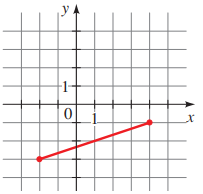# The distance between the pair of points that are graphed below.### Precalculus: Mathematics for Calcu...

6th Edition
Stewart + 5 others
Publisher: Cengage Learning
ISBN: 9780840068071### Precalculus: Mathematics for Calcu...

6th Edition
Stewart + 5 others
Publisher: Cengage Learning
ISBN: 9780840068071

#### Solutions

Chapter 1.8, Problem 12E

(a)

To determine

## To calculate: The distance between the pair of points that are graphed below.Expert Solution

The distance between the pair of points is 210 units

### Explanation of Solution

Given information:

The points are depicted graphically,Formula used:

Distance d(A,B) between two points A=(x1,y1) and B=(x2,y2) in the Cartesian plane is denoted by d(A,B)=(x2x1)2+(y2y1)2 .

Calculation:

Consider the points that are depicted graphically,The two points are A(4,1) and B(2,3) .

Recall that the distance d(A,B) between two points A=(x1,y1) and B=(x2,y2) in the Cartesian plane is denoted by d(A,B)=(x2x1)2+(y2y1)2 .

Evaluate the distance between A(4,1) and B(2,3) .

d(A,B)=(24)2+(3(1))2=36+4=40=210

Thus, the distance between the pair of points is 210 units.

(b)

To determine

### To calculate: The midpoint of the segment that joins the pair of points that are graphed below.Expert Solution

The midpoint of the segment that joins the pair of points is (1,2) .

### Explanation of Solution

Given information:

The points are depicted graphically,Formula used:

Midpoint of the segment that joins two points A=(x1,y1) and B=(x2,y2) in the Cartesian plane is denoted by (x1+x22,y1+y22) .

Calculation:

Consider the points that are depicted graphically,The two points are A(4,1) and B(2,3) .

Recall that the midpoint of the segment that joins two points A=(x1,y1) and B=(x2,y2) in the Cartesian plane is denoted by (x1+x22,y1+y22) .

Evaluate the midpoint of the segment that joins A(4,1) and B(2,3) .

(x1+x22,y1+y22)=(4+(2)2,1+(3)2)=(22,42)=(1,2)

Thus, the midpoint of the segment that joins the pair of points is (1,2) .

### Have a homework question?

Subscribe to bartleby learn! Ask subject matter experts 30 homework questions each month. Plus, you’ll have access to millions of step-by-step textbook answers!